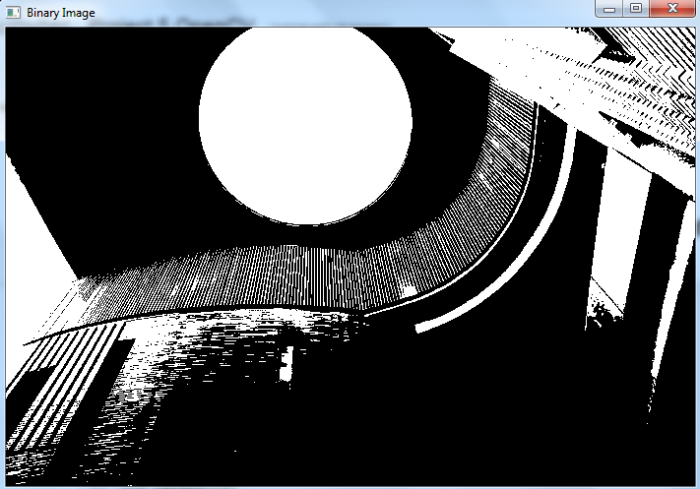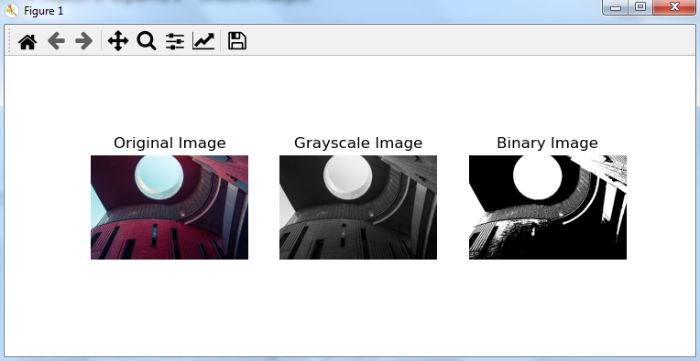# OpenCV Python â€“ How to convert a colored image to a binary image?

We use cv2.threshold() to convert a grayscale image to binary image. To convert a color image to binary image, we first convert the color image to grayscale image using cv2.cvtColor() then apply cv2.threshold() on the grayscale image.

## Steps

One could follow the below given steps to convert a color image to a binary image-

• Import the required library. In all the following examples, the required Python library is OpenCV. Make sure you have already installed it.

• Read an the input image using cv2.imread(). The RGB image read using this method is in BGR format. Optionally assign the read BGR image to img.

• Now convert this BGR image to grayscale image as below using cv2.cvtColor() function. Optionally assign the converted grayscale image to gray.

• Apply thresholding cv2.threshold() on the grayscale image gray to convert it to a binary image. Adjust the second parameter (threshValue) for better binary image.

• Display the converted binary image.

Let's look at some examples for a clear understanding about the question.

We will use the following image as the Input File in the examples below.## Example

In this Python program, we convert a color image to a binary image. We also display the binary image.

# import required libraries
import cv2

# convert the input image to grayscale
gray = cv2.cvtColor(img, cv2.COLOR_BGR2GRAY)

# apply thresholding to convert grayscale to binary image
ret,thresh = cv2.threshold(gray,70,255,0)

# Display the Binary Image
cv2.imshow("Binary Image", thresh)
cv2.waitKey(0)
cv2.destroyAllWindows()


## Output

When you run the above program, it will produce the following output window showing the binary image.## Example

In this example, we convert a color image to a binary image. We also display the original, grayscale and binary images.

# import required libraries
import cv2
import matplotlib.pyplot as plt

# convert the input image to grayscale
gray = cv2.cvtColor(img, cv2.COLOR_BGR2GRAY)

# apply thresholding to convert grayscale to binary image
ret,thresh = cv2.threshold(gray,70,255,0)

# convert BGR to RGB to display using matplotlib
imgRGB = cv2.cvtColor(img, cv2.COLOR_BGR2RGB)

# display Original, Grayscale and Binary Images
plt.subplot(131),plt.imshow(imgRGB,cmap = 'gray'),plt.title('Original Image'), plt.axis('off')
plt.subplot(132),plt.imshow(gray,cmap = 'gray'),plt.title('Grayscale Image'),plt.axis('off')
plt.subplot(133),plt.imshow(thresh,cmap = 'gray'),plt.title('Binary Image'),plt.axis('off')
plt.show()


## Output

When you run the above program, it will produce the following output window showing the original, grayscale and binary images.Notice the difference between the Grayscale image and Binary image. Binary image has only two colors: white and black. The pixel values of the Binary image are either 0 (black) or 255 (white).